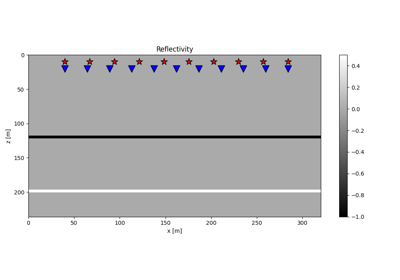# pylops.waveeqprocessing.LSM#

class pylops.waveeqprocessing.LSM(z, x, t, srcs, recs, vel, wav, wavcenter, y=None, kind='kirchhoff', dottest=False, **kwargs_mod)[source]#

Least-squares Migration (LSM).

Solve seismic migration as inverse problem given smooth velocity model vel and an acquisition setup identified by sources (src) and receivers (recs).

Parameters
znumpy.ndarray

Depth axis

xnumpy.ndarray

Spatial axis

tnumpy.ndarray

Time axis for data

srcsnumpy.ndarray

Sources in array of size $$\lbrack 2(3) \times n_s \rbrack$$

recsnumpy.ndarray

Receivers in array of size $$\lbrack 2(3) \times n_r \rbrack$$

vel

Velocity model of size $$\lbrack (n_y \times)\, n_x \times n_z \rbrack$$ (or constant)

wavnumpy.ndarray

Wavelet

wavcenterint

Index of wavelet center

ynumpy.ndarray

Additional spatial axis (for 3-dimensional problems)

kind:str, optional

Kind of modelling operator (kirchhoff, twoway)

dottestbool, optional

Apply dot-test

**kwargs_modint, optional

Additional arguments to pass to modelling operators

Notes

Inverting a demigration operator is generally referred in the literature as least-squares migration (LSM) as historically a least-squares cost function has been used for this purpose. In practice any other cost function could be used, for examples if solver='pylops.optimization.sparsity.FISTA' a sparse representation of reflectivity is produced as result of the inversion.

This routines provides users with a easy-to-use, out-of-the-box least-squares migration application that currently implements:

• Kirchhoff LSM: this problem is parametrized in terms of reflectivity (i.e., vertical derivative of the acoustic impedance - or velocity in case of constant density). Currently, a ray-based modelling engine is used for this case (see pylops.waveeqprocessing.Kirchhoff).

• Born LSM: this problem is parametrized in terms of squared slowness perturbation (in the constant density case) and it is solved using an acoustic two-way eave equation modelling engine (see pylops.waveeqprocessing.AcousticWave2D).

The following table shows the current status of the LSM application:

Kirchhoff integral

WKBJ

Wave eq

Reflectivity

V

X

X

Slowness-squared

X

X

V

Finally, it is worth noting that for both cases the first iteration of an iterative scheme aimed at inverting the demigration operator is a simple a projection of the recorded data into the model domain. An approximate (band-limited) image of the subsurface is therefore created. This process is referred to in the literature as migration.

Attributes
Demoppylops.LinearOperator

Demigration operator operator

Methods

 __init__(z, x, t, srcs, recs, vel, wav, ...) solve(d[, solver]) Solve least-squares migration equations with chosen solver

## Examples using pylops.waveeqprocessing.LSM`#15. Least-squares migration

15. Least-squares migration